Zak transform

Gel'fand mapping,-representation, Weil–Brezin mapping

The Zak transform was discovered by several people in different fields and was called by different names, depending on the field in which it was discovered. It was called the "Gel'fand mapping" in the Russian literature because I.M. Gel'fand [a3] introduced it in his work on eigenfunction expansions associated with Schrödinger operators with periodic potentials. In 1967, almost 17 years after the publication of Gel'fand's work, the transform was rediscovered independently by a solid-state physicist, J. Zak, who called it the "k-q representation" . Zak introduced this representation to construct a quantum-mechanical representation for the motion of a Bloch electron in the presence of a magnetic or electric field [a8], [a9]. It has also been said [a7] that some properties of another version of the Zak transform, called the "Weil–Brezin mapping" in [a1], [a7], were even known to the mathematician C.F. Gauss. Nevertheless, there seems to be a general consent among experts in the field to call it the Zak transform, since Zak was indeed the first to systematically study that transform in a more general setting and recognize its usefulness.

The Zak transform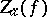of a functionis defined by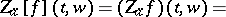(a1)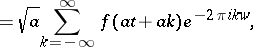where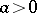andandare real. When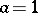, one denotesby.

Ifrepresents a signal, then its Zak transform can be considered as a mixed time-frequency representation of, and it can also be considered as a generalization of the discrete Fourier transform ofin which an infinite sequence of samples in the form,, is used (cf. also Fourier transform).

Examples.

Ifandoutside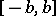,, then,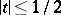.

The Zak transform of the Gaussian functionis easily shown to bewhere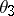is the third theta-function, defined by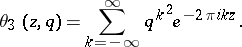Existence.

Ifis integrable or square integrable (cf. Integrable function), its Zak transform exists almost everywhere. In particular, ifis a continuous function such that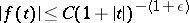, for some, for all, then its Zak transform exists and defines a continuous function.

Elementary properties.

1) (linearity): for any complex numbersand,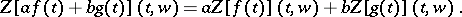2) (translation): for any integer,in particular,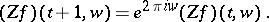3) (modulation):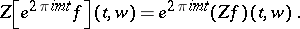4) (periodicity): The Zak transform is periodic in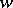with period one, that is,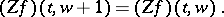5) (translation and modulation): By combining 2) and 3) one obtains6) (conjugation):7) (symmetry): Ifis even (cf. also Even function), thenand ifis odd, then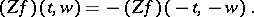From 6) and 7) it follows that ifis real-valued and even, then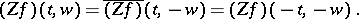Because of 2) and 4), the Zak transform is completely determined by its values on the unit square.

8) (convolution): Let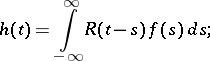then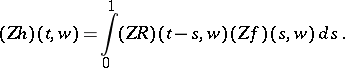Analytic properties.

Ifis a continuous function such thatasfor some, then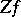is continuous on. A rather peculiar property of the Zak transform is that ifis continuous, it must have a zero in. The Zak transform is a unitary transformation fromonto; see [a10], p. 481.

Inversion formulas.

The following inversion formulas for the Zak transform follow easily from the definition, provided that the series defining the Zak transform converges uniformly (cf. also Uniform convergence):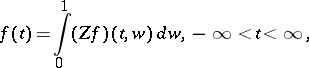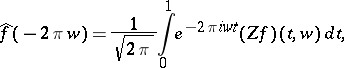and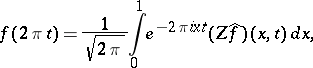where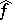is the Fourier transform of, given byApplications.

The Zak transform has been used successfully in various applications in physics, such as in the study of the coherent states representation in quantum field theory [a6], and in electrical engineering, such as in time-frequency representation of signals and in digital data transmission; see [a4], [a5].

The applications of the Zak transform are not limited to only physics and engineering. More recent applications of it in mathematics have proved to be very useful; in particular, to simplify proofs of some important results. A case in point is the Gabor representation problem. The Gabor representation problem can be stated as follows: Given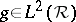and two real numbers,,different from zero, is it possible to represent any functionby a series of the form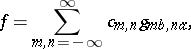whereare the Gabor functions, defined by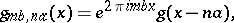and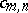are constants? And under what conditions is the representation unique?

The Zak transform has been used successfully to study the orthogonality and the completeness of Gabor frames in the crucial case where; see [a2], [a10].